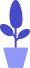# Coordinate system and color of Python Pygame

Estimated reading: 2 minutes 59 views

In the previous tutorial, we have learned about Python Pygame, and now we will continue to learn to use Python Pygame to draw graphics.

## Coordinate system of Python Pygame

Before we dive into Pygame, we need to take a look at the difference between Pygame and turtle drawing in coordinate systems. In the turtle drawing, the origin is at the center of the screen, the farther to the right of the screen, the larger the x-coordinate, and the further up the screen, the larger the y-coordinate.

Pygame uses a new coordinate system, the more common window-oriented coordinate system. The upper left corner of the Pygame window is the origin (0, 0). The x-coordinate still gets bigger as we move to the right, however, the x-coordinate has no negative values. As you move down, the value of the y-coordinate gradually increases, and the y-coordinate has no negative values.

Suppose we have a Surface object that is 10 pixels wide and 10 pixels high, as shown in Figure 1.figure 1
We can locate coordinates by specifying integers for the X and Y axes, represented as a tuple of two integers, such as (5,2) and (4,6). The 1st integer is the X coordinate and the 2nd integer is the Y coordinate. We can display the pixel at the 1st coordinate as red and the pixel at the 2nd coordinate as yellow, as shown in Figure 2.figure 2

## Colors for Python Pygame

In Pygame, we use RGB color values ​​to represent colors. We have introduced RGB values ​​before and will not repeat them here. For example, we could use the tuple (0, 0, 0) for black, (255, 255, 255) for white, (255, 0, 0) for red, (0, 255, 0) for green, and (0) , 0, 255) for blue.

We don’t want to rewrite a tuple of 3 numbers every time we want to use a specific color in our program, so we’ll create constants to hold these tuples and use the colors that these tuples represent to name the constant:

``````BLACK=(0,0,0)
WHITE=(255,255,255)
GREEN=(0,255,0)
BLUE=(0,0,255)
RED=(255,0,0)``````